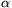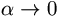Középiskolai Matematikai és Fizikai Lapok
Informatika rovattal
 Már regisztráltál? Új vendég vagy?

# Exercises and problems in Physics April 1998

## New experimental problem:

m. 197. Examine how the electric power output of the dynamo of a bicycle through different constant resistances depends on the number of revolutions of the rotor.

## New exercises:

FGy. 3150. A row-boat travels downstream on a river at a speed of 8 m/s with respect to the shore. A motor-boat comes from the opposite direction at a speed of 10 m/s with respect to the water. 10 seconds after meeting each other they are at 160 metres from each other. At what speed does the river flow?

FGy. 3151. After disassembling a new type energy sparing light source one can see a 0.25 watt, 27 ohm resistor and a complicated circuit (including the part that emits the light) connected in series inside it. The new light source gives out as much light as a 60 watt filament lamp. By at least how many percents is its power consumption reduced?

FGy. 3152. When a tank vehicle half filled with some liquid is in uniform acceleration what is the direction of the upthrust inside it?

FGy. 3153. A piece of -10 oC ice is heated to -1 oC using a certain quantity of energy. Then another 20 times as much energy is necessary to finally obtain water. Using that the specific heat of ice is half of the specific heat of 4.2 kJ/(kg oC) of water, determine the heat of fusion of ice from the above measurement data.

FGy. 3154. A pair of compasses hangs on a thread fastened to one of its ends. At what angle should the compasses be open so that their joint is at the highest possible position? What is the position of the lower leg then? (The legs should be approximated as homogeneous rods.)

## New problems:

FF. 3155. Estimate the radius of a drop of water in which the density of the water has increased by one per mil due to the pressure of curvature. (The compressibility of water is 5.10-10 Pa-1).

FF. 3156. A satellite is launched from a spherical planet in such a way that its velocity vector at its return to the planet is parallel to its velocity vector at launching. The angular distance between the launching and the landing point is. How much time does the satellite spend in space if the period of a satellite on a circular orbit near the surface of the planet is T0? What is the maximum distance of the satellite from the surface of the planet if the radius of the planet is R? Examine the limiting case when.

FF. 3157. There are diodes that do not conduct under 2.5 V, while at 2.5 V only the internal resistance of the current supply limits the current flowing through them, and they emit light (LEDs). Design a simple circuit with the following properties using two of these diodes and different ohmic resistors: when a voltage between 4.25 V and 5.5 V is connected to the circuit then only one of the diodes lights up, when a voltage between 5.5 V and 6 V is connected to the circuit then both diodes light up, and when a voltage under 4.25 V is connected to the circuit then none of the LEDs lights up. The current flowing through the diodes must not be higher than 25 mA.

FF. 3158. The end of an optical fibre is cut perpendicularly to the fibre. What is the minimum required refractive index so that light inciding from any direction at the end of the fibre can enter the fibre but it cannot leave the fibre on the side?